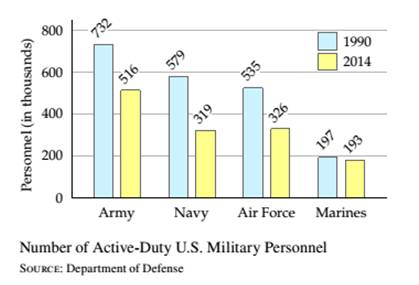Chapter 9.3, Problem 30ESMathematical Excursions (MindTap C...

4th Edition
Richard N. Aufmann + 3 others
ISBN: 9781305965584

Solutions

Chapter
SectionMathematical Excursions (MindTap C...

4th Edition
Richard N. Aufmann + 3 others
ISBN: 9781305965584
Textbook Problem

The graph below shows the number of active-duty U.S. military personnel, in thousands, in 1990 and 2014 . Which branch of the military had the greatest percent decrease in personnel from 1990 to 2014 ? What was the percent decrease for this branch of the service? Round to the nearest tenth of a percent.Number of Active-Duty U.S. Military PersonnelSOURCE: Department of Defense

To determine

The military branch which had the greatest percent of decrease in personnel from 1990 to 2014

Explanation

Given Information:

The graph shows the number of active-duty US military personnel in thousands from year 1990 to 2014

Calculation:

Calculate the decrease in active duty US military personnel of Army

Decrease in number of members from 1990 to 2014 ,

732516=216

Apply basic percent equation formula

PB=A

Here, P (percent) is unknown, B(base) is the number active Army personnel in year 1990 , 732 and A(amount) is the number of active ARMY personnel decreased till 2014 , 216

P×732=216P=216732P=0.295

Thus, percent decrease in US Army personnel from 1990 to 2014 is 29.5%

Calculate the decrease in active duty US military personnel of Navy,

Decrease in number of members from 1990 to 2014 ,

579319=260

Apply basic percent equation formula

PB=A

Here, P (percent) is unknown, B(base) is the number of active Navy personnel in year 1990 , 579 and A(amount) is the number of active Navy personnel decreased till 2014 , 260

P×579=260P=260579P=0.449

Thus, percent decrease in active Navy personnel from 1990 to 2014 is 44

Still sussing out bartleby?

Check out a sample textbook solution.

See a sample solution

The Solution to Your Study Problems

Bartleby provides explanations to thousands of textbook problems written by our experts, many with advanced degrees!

Get Started

Solve the equations in Problems 1-6

Mathematical Applications for the Management, Life, and Social Sciences

In Exercises 7-10, find the slope of the line shown in each figure. 7.

Applied Calculus for the Managerial, Life, and Social Sciences: A Brief Approach

Evaluate the integral. 21. 00.6x2925x2dx

Single Variable Calculus: Early Transcendentals